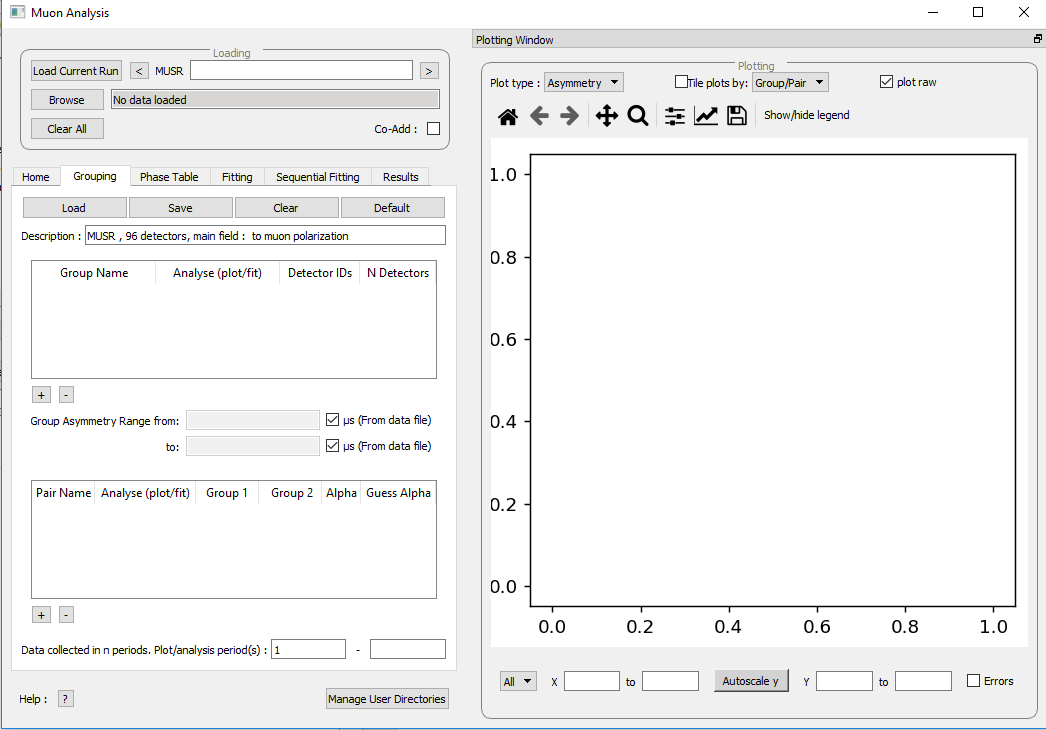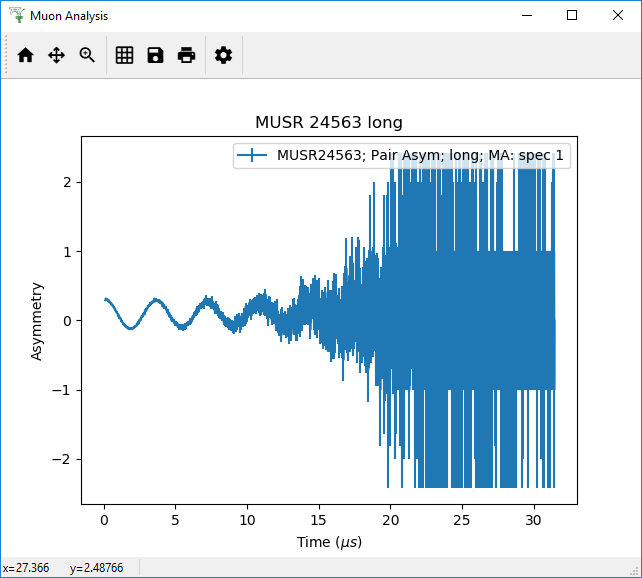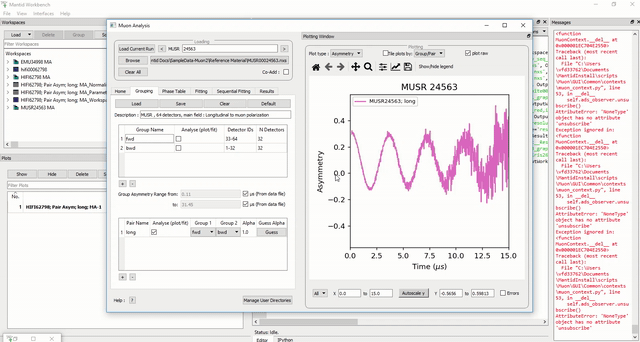$$\renewcommand\AA{\unicode{x212B}}$$

# The Tabs - Grouping¶

## Grouping¶

The Grouping tab allows:

• Grouping files to be loaded, saved, modified or cleared.

• Regrouped data to be plotted.

• $${\alpha}$$ values to be determined from T20 measurements.

• Raw data plotting options to be selected.

The Grouping options are shown belowFigure 18: The Grouping Options tab of the Muon Analysis Interface.¶

Standard detector groupings are usually loaded when a data file is opened, which each incorporate one half of the total detectors of the equipment being used. For example, for the EMu spectrometer detectors 1 – 48 and 49 - 96 are automatically assigned to the forward and backward detector arrays.

### Data Grouping¶

When a workspace is opened using the Muon Analysis GUI, a plot of the data automatically opens with it. For measurements not taken in transverse fields, this plot is set to show the asymmetry by default, which is the normalised ratio of the Forward ($$F$$) and Backward ($$B$$) detector banks’ positron counts; as given by the equation:

(1)$P_z(t) = A_0G_z(t) = \frac{F(t) - \alpha B(t)}{F(t) + \alpha B(t)}$Figure 19: The default plot window for MUSR00024563 showing raw (unbinned) data for the long pair asymmetry.¶

Data collected only in the Forward, or Backward, detector banks can be viewed in the plotting window of the Muon Analysis GUI.

1. Load the MUSR00024563 file in the Muon Analysis GUI.

2. On the Grouping Tab tick the checkboxes next to forward (fwd) and backward (bwd) detector arrays. Then untick the checkbox next to long.

3. Observe and consider the difference in the plot. This is shown in Figure 18.

### Plot Type¶

Though the plot type shows asymmetry by default it can be changed to show positron counts against time using the Plot Type drop-down menu, which can be found in the Plotting section of the Home tab. To demonstrate this, follow the example below.

2. Ensure that the Group / Pair drop-down menu is set to either ‘bwd’ or ‘fwd’, as the plot type will not change if this is set to ‘long’.

3. Underneath the Plot Data section, in the Plot Type box change the type from ‘Asymmetry’ to ‘Counts’. There should be a change in the data plot. See Figure 21 for the process.Figure 21: How to change the plot type using the Muon Analysis GUI.¶

Plot options (such as symbol type, lines etc.) are described in Overlaying and Styling Plots section of Other Mantid Functions and Basic Data Manipulation.

In addition, should data have been collected using differed timing periods (as one would during an RF experiment, for example), the data collected during each separate time period can also be viewed separately (again for either the Forward and Backward detector arrays) by adjusting the period number.

When plotting data according to (1) the default alpha value is 1. An accurate alpha value can be determined using the Guess Alpha option found in the Grouping tab.

### Adding Detector Groups and Pairs¶

Different detector grouping configurations can be entered manually, as can Group Pairs. A Group Pair is a combination of two different detector groups. For example, the default detector groups fwd and bwd are used a pair called long. This combines the detectors from both groups in order to be able to view them together. For the MuSR equipment this default case means viewing all 64 detectors. A detector group pair can include any two groups found in the group table.

To try assigning new detector groups and pairs with the MUSR00024563 file (see Home for how to load files), follow the instructions below. For assistance, see figures 22 and 23.

1. First, go to the Grouping tab in the muon analysis GUI.

2. Under the ‘Description:’, there is a table of the existing groups, with Group Name, Analyse (plot/fit), Detector IDs and N Detectors as columns. bwd and fwd should already be saved as groups.

3. In the blank space under the existing group names, right click, select Add Group and enter the name sample_fwd. This will be one of our new groups.

4. Assign this new group Detector IDs of 1-2, in the blank space in the same row. Ndet will then fill automatically.

5. Repeat this process to also add a group called sample_bwd with Detector IDs of 10-11.

NB any string of numbers can be defined as Detector IDs, so long as they are within the number of detectors on the equipment used (i.e. 1,3-5,15,17-18 would be valid and 1,3-5,10324 would be invalid).

1. At the bottom of the tab there should be a table containing the default long pair details. The columns in this table read Pair Name, Analyse (plot/fit), Group 1, Group 2, Alpha, and Guess Alpha.

2. In the blank space under long in the Name column, right click and enter a name for a new pair, such as sample_long.

3. In the same row as the new pair, in the Group 1 column select sample_fwd from the drop down menu and in the Group 2 column select sample_bwd. To update the:math:{alpha} value, click Guess Alpha. This process is shown in figure 21 below.

Finally, $${\alpha}$$ can be determined for any given detector pairing (via the analysis of transverse field data) by clicking on Guess Alpha. Click on a specific Group Pair name to select it. The $${\alpha}$$ value column is automatically updated. The value in the column is applied to all subsequent data reduction when asymmetry plots are desired. The detector calibration factor, $${\alpha}$$ is described in more detail in The Basics of Data Reduction.